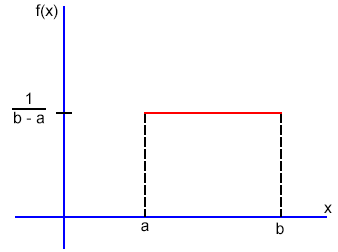# Continuous uniform distributions

hi… if a probability is uniformly distributed from 27 to 89… the total number of outcomes … is (89-27 = 62 or 89-27+1 = 63) cuz if you include 27 … you get 63 out comes not 62… similarly… 34 to 38 … is 5 outcomes… 34,35,36,37,38… so the probability for 34 to 38 should be 5 /63… … i know i am wrong cuz the answer is 4 /62… but can you guys tell me why i am wrong…

Um . . . the total number of outcomes is (uncountably) infinite. This is a continuous distribution, not just integers.

3 Likes

I would look at the continuous uniform distribution as if it was just a rectangle. Therefore, finding the probability is just finding the area of it.

• Rectangle Area = Base x Height
• Uniform Distribution = (b-a) * f(x) , total area must be 1.
f(x) = \frac{1}{(b-a)}To find your ans is simply just applying the formula:

P({34}\leq{x}\leq{38}) = {(38-34)}\times{\frac{1}{(89-27)}}

For the uniform distribution, f(x) is constant over the possible value of x.

2 Likes